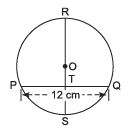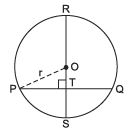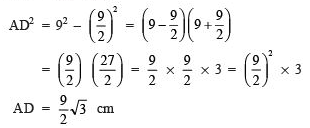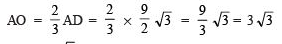Courses

# Long Answer Type Questions- Circles Class 9 Notes | EduRev

## Class 9 : Long Answer Type Questions- Circles Class 9 Notes | EduRev

The document Long Answer Type Questions- Circles Class 9 Notes | EduRev is a part of the Class 9 Course Class 9 Mathematics by VP Classes.
All you need of Class 9 at this link: Class 9

Question 1. Look at the adjoining figure. If O is the centre of the circle and ST = 3 cm, then find the radius of the circle when RS ⊥ PQ.
Solution
: Let us join O and P such that OP = r.
∵ RS ⊥ PQ
∴ T is the mid-point of PQ.⇒ PT = (1/2) PQ
⇒ PT = (1/2) x 12 cm = 6 cm                 [∵ PQ = 12 cm      (Given)]
And ∠ OTP = 90°
Also OS = r and TS = 3 cm
∴ OT = OS - TS = (r - 3) cm
Now, in right ΔOTP, we have, OP2 = PT2 + OT2
⇒ r= 62 + (r - 3)2
⇒ r2 = 36 + r+ 9 -  6r
⇒ 6r = 45⇒ r =(45/6) = (15/2) = 7.5 cm
Thus, the radius of the circle is 7.5 cm.

Question 2. An equilateral triangle is inscribed in a circle. Find the radius of the circle.
Solution:
Let ABC be an equilateral triangle such that AB = BC = AC = 9 cm                  (each)
Let us draw a median AD corresponding to BC.
∴ BD =(1/2) BC
⇒ BD = (1/2) x 9 cm = (9/2)cm
Also, AD ⊥ BC                  [∵ O is the centre of the circle]
Now, in right ΔADB,AD2 = AB2 - BD2Since, in an equilateral triangle, the centroid and circumcentre coincide.

∴ AO: OD = 2:1

⇒⇒ Radius = 3√3 cm

Thus, the required radius =  3√3 cm

Offer running on EduRev: Apply code STAYHOME200 to get INR 200 off on our premium plan EduRev Infinity!

125 docs

,

,

,

,

,

,

,

,

,

,

,

,

,

,

,

,

,

,

,

,

,

;